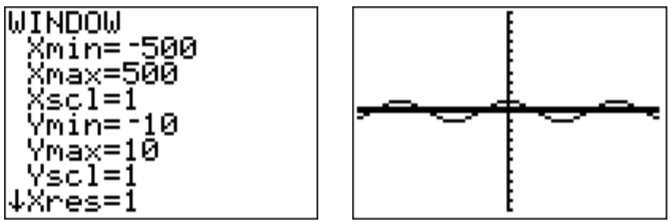# 26.9: A.9- Common errors

$$\newcommand{\vecs}{\overset { \rightharpoonup} {\mathbf{#1}} }$$ $$\newcommand{\vecd}{\overset{-\!-\!\rightharpoonup}{\vphantom{a}\smash {#1}}}$$$$\newcommand{\id}{\mathrm{id}}$$ $$\newcommand{\Span}{\mathrm{span}}$$ $$\newcommand{\kernel}{\mathrm{null}\,}$$ $$\newcommand{\range}{\mathrm{range}\,}$$ $$\newcommand{\RealPart}{\mathrm{Re}}$$ $$\newcommand{\ImaginaryPart}{\mathrm{Im}}$$ $$\newcommand{\Argument}{\mathrm{Arg}}$$ $$\newcommand{\norm}{\| #1 \|}$$ $$\newcommand{\inner}{\langle #1, #2 \rangle}$$ $$\newcommand{\Span}{\mathrm{span}}$$ $$\newcommand{\id}{\mathrm{id}}$$ $$\newcommand{\Span}{\mathrm{span}}$$ $$\newcommand{\kernel}{\mathrm{null}\,}$$ $$\newcommand{\range}{\mathrm{range}\,}$$ $$\newcommand{\RealPart}{\mathrm{Re}}$$ $$\newcommand{\ImaginaryPart}{\mathrm{Im}}$$ $$\newcommand{\Argument}{\mathrm{Arg}}$$ $$\newcommand{\norm}{\| #1 \|}$$ $$\newcommand{\inner}{\langle #1, #2 \rangle}$$ $$\newcommand{\Span}{\mathrm{span}}$$$$\newcommand{\AA}{\unicode[.8,0]{x212B}}$$

The following shows many common errors and mistakes that may produce a false result or an error. If none of the items below help to resolve your problem, then you can also reset the calculator to factory setting (see section [SEC A.10:resetting-the-calculator]).

## Reset window to standard window

Many problems with graphing can be resolved by changing the window back to the standard window. To do so, press $$\boxed{\text{zoom}}$$$$\boxed{\text{6}}$$.Note, that the standard window has the following settings:## PLOT marked

Sometimes an error is produced when graphing a function due to having any of the PLOTs marked in the ‘Y=’ menu.Make sure that all of the PLOTs are unmarked!

## Errors in graphing functions

Graph the functions $$y=\ln(x-3)$$ or $$y=\dfrac{1}{x^3-9x}$$.The calculator graphic is not really the graph, but has extra parts or missing parts of the graph (depending on the version of the calculator: TI-83 is much worse than TI-84). The reason for this is that the calculator just approximates the graph pixel by pixel and does not represent the exact graph. In particular, you should not just copy the graph from the calculator onto your paper, but interpret what you see and draw the interpreted graph!

## Fractions need parenthesis for numerator and denominator

When entering a rational function, (or any fraction), the numerator and denominator has to be entered with parenthesis. For example, $$y=\dfrac{x-3}{x^2-1}$$ is entered as follows.Trigonometric functions should generally be graphed in radians. For example, the cosine function in radian gives the following.On the other hand, the cosine in degrees displays as follows.The reason is that one period is now $$360$$ on the $$x$$-axis. Rescaling the calculator to a wider $$x$$-scale shows the effect more clearly.The calculator also gives different values for the trigonometric functions in degrees or radians. This is shown below.## Table setup

The table can be setup to generate a list of outputs, or to take an input value and generate its output value. This depends on the independent variable in the TABLE SETUP being set to ‘Auto’ or ‘Ask,’ respectively.## Using the wrong minus sign

Often a syntax error is due to using the minus sign $$\boxed{\text{-}}$$ instead of $$\boxed{\text{(-)}}$$. Note that $$\boxed{\text{-}}$$ is used to subtract two numbers, whereas $$\boxed{\text{(-)}}$$ gives the negative of a number.

For example, to calculate $$-3-5$$, press $$\boxed{\text{(-)}}$$$$\boxed{\text{3}}$$$$\boxed{\text{-}}$$$$\boxed{\text{5}}$$.This page titled 26.9: A.9- Common errors is shared under a CC BY-NC-SA 4.0 license and was authored, remixed, and/or curated by Thomas Tradler and Holly Carley (New York City College of Technology at CUNY Academic Works) via source content that was edited to the style and standards of the LibreTexts platform; a detailed edit history is available upon request.